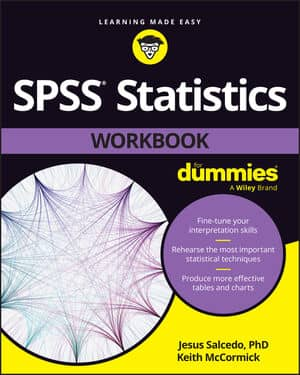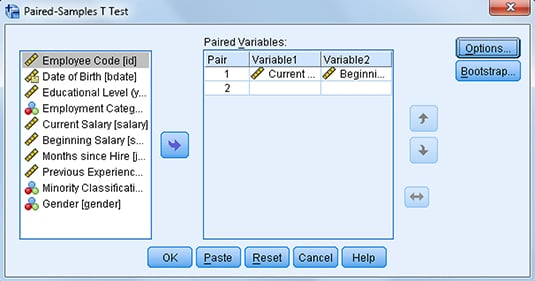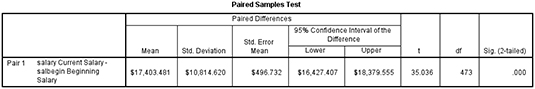##### SPSS Statistics Workbook For DummiesThe Paired-Samples T Test in SPSS Statistics determines whether means differ from each other under two conditions. For example, you can use this test to assess whether there are mean differences when the same group of people have been assessed twice, such as when determining if an intervention had an impact by using a before and after design.

The use of the Paired-Samples T Test is not limited to situations in which a group of people have been assessed at two time points. You can also use this test when a group has been assessed only once. For example, a group may have been asked to provide ratings on two products; in this situation, researchers can determine if one product was rated higher (preferred) than the other.

The following figure shows the Paired-Samples T Test dialog box. Here, you can determine the change between current salary and beginning salary; so, in this example, you have data for one group of people that have been assessed twice (once when they were hired [beginning salary]) and currently [current salary]).Completed Paired-Samples T Test dialog box.

In the next figure, under the Mean column, you can see the mean difference in salary between current and beginning salary. In this case, you can see that the mean difference between the two conditions is \$17,403.81.

You now need to determine if the difference between the means is significantly different or if the difference you’re seeing is just due to chance. The T column displays the actual result of the T Test and the DF column tells IBM SPSS Statistics how to determine the probability of the T statistic. The Sig. (2-Tailed) column tells the probability of the null hypothesis being correct.

In the case of the Paired-Samples T Test, the null hypothesis is that the two means are equal; the alternative hypothesis is that the two means differ. If the probability value of the null hypothesis is very low (less than 0.05), you can conclude that the means are significantly different from each other.

In the following figure, you can see that there are significant differences between the means, so employees are earning significantly more income now than when they first started working for this company.Paired Samples Test table.